# Python Program to perform cross join in Pandas

• Last Updated : 10 Jul, 2020

In Pandas, there are parameters to perform left, right, inner or outer merge and join on two DataFrames or Series. However there’s no possibility as of now to perform a cross join to merge or join two methods using `how="cross"` parameter.

Cross Join :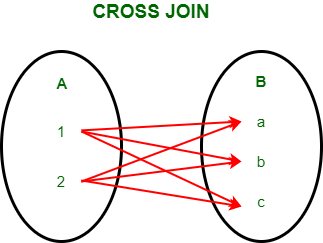Example 1:

The above example is proven as follows

 `# importing pandas module``import` `pandas as pd ``  ` `# Define a dictionary with column A``data1 ``=` `{``'A'``: [``1``, ``2``]} ``    ` `# Define another dictionary with column B``data2 ``=` `{``'B'``: [``'a'``, ``'b'``, ``'c'``]}  ``  ` `# Convert the dictionary into DataFrame  ``df ``=` `pd.DataFrame(data1, index ``=``[``0``, ``1``])``  ` `# Convert the dictionary into DataFrame  ``df1 ``=` `pd.DataFrame(data2, index ``=``[``2``, ``3``, ``4``]) `` ` `# Now to perform cross join, we will create``# a key column in both the DataFrames to ``# merge on that key.``df[``'key'``] ``=` `1``df1[``'key'``] ``=` `1`` ` `# to obtain the cross join we will merge ``# on the key and drop it.``result ``=` `pd.merge(df, df1, on ``=``'key'``).drop(``"key"``, ``1``)`` ` `result`

DataFrame 1: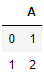DataFrame 2 :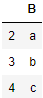Output :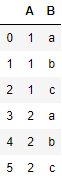Example 2:

Cross join on two DataFrames for user and product.

 `# importing pandas module``import` `pandas as pd ``  ` `# Define a dictionary containing user ID``data1 ``=` `{``'Name'``: [``"Rebecca"``, ``"Maryam"``, ``"Anita"``],``        ``'UserID'``: [``1``, ``2``, ``3``]} ``    ` `# Define a dictionary containing product ID ``data2 ``=` `{``'ProductID'``: [``'P1'``, ``'P2'``, ``'P3'``, ``'P4'``]} ``  ` `# Convert the dictionary into DataFrame  ``df ``=` `pd.DataFrame(data1, index ``=``[``0``, ``1``, ``2``])``  ` `# Convert the dictionary into DataFrame  ``df1 ``=` `pd.DataFrame(data2, index ``=``[``2``, ``3``, ``6``, ``7``]) `` ` `# Now to perform cross join, we will create``# a key column in both the DataFrames to ``# merge on that key.``df[``'key'``] ``=` `1``df1[``'key'``] ``=` `1`` ` `# to obtain the cross join we will merge on ``# the key and drop it.``result ``=` `pd.merge(df, df1, on ``=``'key'``).drop(``"key"``, ``1``)`` ` `result`

DataFrame 1: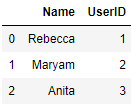DataFrame 2 :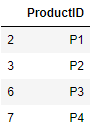Output :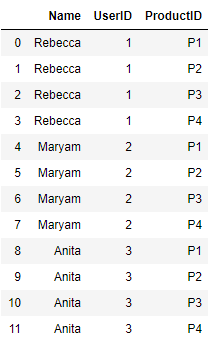Example 3:

 `# importing pandas module``import` `pandas as pd ``  ` `# Define a dictionary with two columns``data1 ``=` `{``'col 1'``: [``0``, ``1``],``        ``'col 2'``: [``2``, ``3``]} ``    ` `# Define another dictionary ``data2 ``=` `{``'col 3'``: [``5``, ``6``],``        ``'col 4'``: [``7``, ``8``]}  ``  ` `# Convert the dictionary into DataFrame  ``df ``=` `pd.DataFrame(data1, index ``=``[``0``, ``1``])``  ` `# Convert the dictionary into DataFrame  ``df1 ``=` `pd.DataFrame(data2, index ``=``[``2``, ``3``]) `` ` `# Now to perform cross join, we will create``# a key column in both the DataFrames to``# merge on that key.``df[``'key'``] ``=` `1``df1[``'key'``] ``=` `1`` ` `# to obtain the cross join we will merge on ``# the key and drop it.``result ``=` `pd.merge(df, df1, on ``=``'key'``).drop(``"key"``, ``1``)`` ` `result`

DataFrame 1: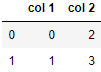DataFrame 2 :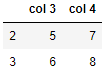Output :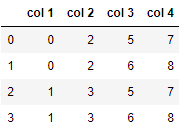My Personal Notes arrow_drop_up